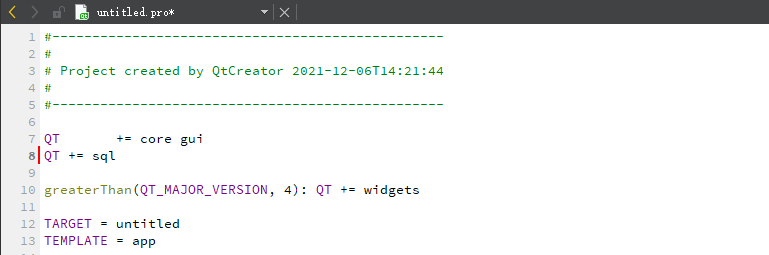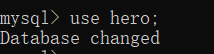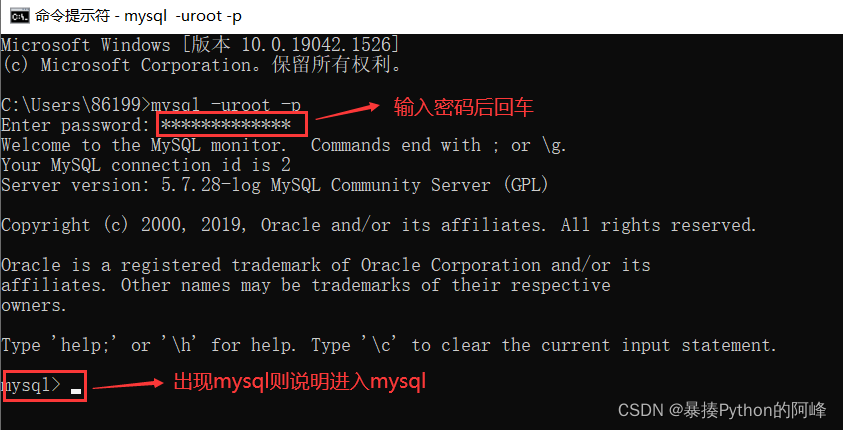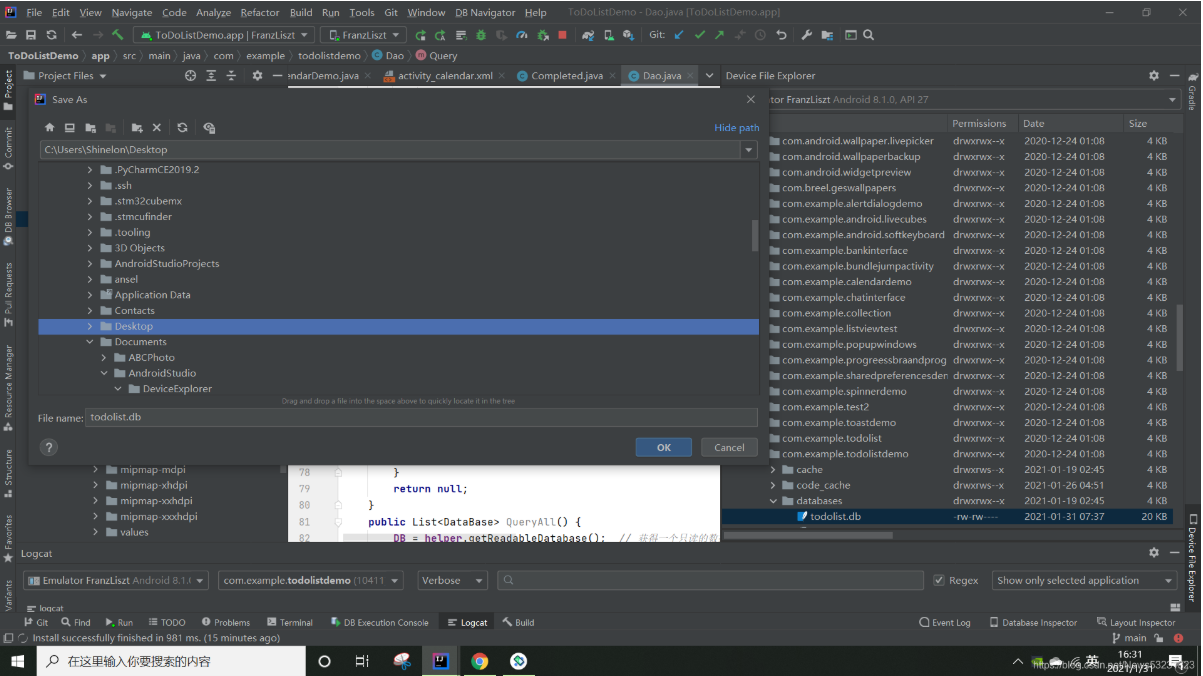# ThinkPHP 的模型使用对数据库增删改查（五）

+关注继续查看

ThinkPHP 的模型使用

// 直接连接数据库，但是得先去配置文件中配置下才行
class IndexAction extends Action {
//对应数据库的表 ,一定要对应数据库的表名  大小没关系
$user =new Model('Leyangjun'); //$user = M('Leyangjun');  //等同上面的new Model()
$arr =$user ->select();
var_dump($arr); } //对数据库的 增删改查 操作（简称：CURD） 一：增 -C Create$m->add()
$m=new Model('User');$m=M('User');
$m->字段名='值'; //$user->name='yangjun';
$m->add(); //返回值是新增的id号 二：删 -D Delete$m->delete()
$m=M('User');$m->delete(2);               //删除id为2的数据,如果不写2，就会删除全部数据
$count =$m->where('id=2')->delete(); //与上面效果相同，也是删除id为2的数据
//返回值是受影响行数
if($count >0){$this->success("数据删除成功！")；
}else{
} $this->error("数据删除失败！")； 三：改 -U Update$m->save()
$m=M('User');$data['id']=1;
$data['name']='leyangjun'; //将id=1的name值改成leyangjun$m->save($data); //返回值是受影响行数 四：查 -R Read$m->select()
$m=new Model('User'); ==$m=M('User');
1：select
$m->select(); //获取所有数据，以数组形式返回 2：find$m->find($id); //获取单条数据 默认取的是id=1的数据，想取第二条find(2) 3：getField(字段名) //获取一个具体的字段值$arr = $m->getField('name'); //获取一个具体的字段值,默认读取的是第一条的name$arr=\$m->where('id=2')->getField('username'); //连贯操作，去id=2的username值

}Python编程：MySQLdb模块对数据库的基本增删改查操作
Python编程：MySQLdb模块对数据库的基本增删改查操作
56 039 0C/C++ Qt 数据库QSql增删改查组件应用
Qt SQL模块是Qt中用来操作数据库的类，该类封装了各种SQL数据库接口，可以很方便的链接并使用，数据的获取也使用了典型的Model/View结构，通过MV结构映射我们可以实现数据与通用组件的灵活绑定，一般SQL组件常用的操作，包括，读取数据，插入数据，更新数据，删除数据，这四个功能我将分别介绍它是如何使用的。
89 0MySQL数据库基础学习Day1：创建库与表（数据库操作、表操作、数据操作：增删改查）2
MySQL数据库基础学习Day1：创建库与表（数据库操作、表操作、数据操作：增删改查）
49 0MySQL数据库基础学习Day1：创建库与表（数据库操作、表操作、数据操作：增删改查）
MySQL数据库基础学习Day1：创建库与表（数据库操作、表操作、数据操作：增删改查）
66 0126 0Yii2框架（二）数据库相关操作
Yii2.0大概封装了两大众方法来执行数据库操作： 1：query()和queryXXX()方法，query()方法返回的是对象一般用的较少，当然，这个是对我来说，具体看你自己的需求。 2：execute方法
84 097 0ThinkPHP框架学习(含数据库及I函数)

1244 0498322

ORACLE 10g 数据库体系结构图